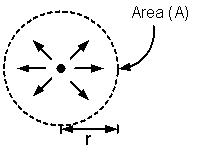×#### Thank you for registering.

One of our academic counsellors will contact you within 1 working day.

Click to Chat

1800-1023-196

+91-120-4616500

CART 0

• 0

MY CART (5)

Use Coupon: CART20 and get 20% off on all online Study Material

ITEM
DETAILS
MRP
DISCOUNT
FINAL PRICE
Total Price: Rs.

There are no items in this cart.
Continue Shopping• Complete Physics Course - Class 11
• OFFERED PRICE: Rs. 2,968
• View Details

```How to Determine the Photoelectric Current

Let P be the power of a point source of electromagnetic radiations, then intensity I at distance r from the source is given by

I = P/4πr2 (W/m2)

If A is the area of a metal surface on which radiations are incident, then the power received by the plate is

P' = IA = P/4πr2 (W)

If f is the frequency of radiation, then the energy of photon is given by

E = hfThe number of photons incident on the plate per second (called photon flux) is given by

Φ  = P' / E = [P/4πr2 × A / hf]

If f > f0 (threshold frequency) and photon efficiency of the metal plate is η%, then the number of photoelectrons emitted per second is given by

n = Φη/100 [P/4πr2 × A / hf]] η/100

Finally, the photocurrent i is given by

i = ne

Where e is the charge of an electron (e = 1.6 × 10–19 J)

Illustration 1:

Photoelectric threshold of metallic silver is λ = 3800 Å. Ultra-violet light of λ = 2600 Å is incident on silver surface. Calculate

(i)     the value of work function in joule and in eV.

(ii)    maximum kinetic energy of the emitted photoelectrons.

(iii)    the maximum velocity of the photo electrons (mass of the electron = 9.11 x 10–31 kg)

Solution:

(i)     I0 = 3800 Å

W = hf0 = h c/λ0 = 6.633 × 10–34 × 3 × 108 / 3800 × 10–10

J = 6.63 × 10–34 × 3 × 108 / 3800 × 10–10    J = 5.23 × 10–19        J = 3.27 eV

(ii)    Incident wavelength λ = 2600 Å

f = incident frequency = 3 × 108 / 2600 × 10–10 Hz

Then Tmax = hf – W0

hf = 6.63 × 1034 × 3 × 108 / 2600 × 10–10 = 6.63 × 3 / 2.6 × 10–19 J = 7.65 × 10–19 J = 4.78 eV

Tmax = hf – W0 = 4.78 eV – 3.27 eV = 1.51 eV.

(iii)  Tmax = 1/2 mv2max

∴ vmax = √2Tmax / m = √2 × 2.242 × 10–19 / 9.11 × 10–31 = 0.7289 × 106 ms–1

Illustration 2:

Sun gives light at the rate of 1400 Wm–2 of area perpendicular to the direction of light. Assume λ(sun light) = 6000 Å. Calculate the

(a)    number of photons/sec arriving at 1m2 area at that part of the earth, and

(b)    number of photons emitted from the sun/sec assuming the average radius of Earth's orbit is 1.49 x 1011 m.

Solution:

I = 1400 W / m2;       I = 6000 A

(a)    n/sec = A / E/photon = (1400 × 1) × (6000 × 10–10) / 6.63 × 10–34 × 3 × 108 = 4.22 × 1021

(b)    n/sec = Power of Sun(W) / E/photon = I × (4R2) × (6000 × 10–10) / 6.63 × 10–34 × 3 × 108 = 1.178 × 1045

Illustration 3:

An isolated hydrogen atom emits a photon of 10.2 eV. Calculate:

(a)    momentum of photon emitted

(b)    recoil momentum of the atom

(c)    kinetic energy of the recoil atom. Mass of proton = Mp = 1.67 x 10–27 kg.

Solution:

(a)    momentum (p) = E/c

=> p = 10.2 × 1.6 × 10–19 / 3 × 108 = 5.44 × 10–27 kg m/s

(b)    recoil momentum of atom = p (Law of conservation of momentum)

=> recoil momentum = 5.44 x 10–27 kg m/s

(c)    KE = 1/2 mv2 (v = recoil speed of atom, m = mass of H-atom)

=> KE = 1/2 (p/m)2 = p2/2m

=> KE = (5.44 × 10–27)2 / (2 × 1.67 × 10–27) = 8.86 × 10–27 J
```### Course Features

• 101 Video Lectures
• Revision Notes
• Previous Year Papers
• Mind Map
• Study Planner
• NCERT Solutions
• Discussion Forum
• Test paper with Video Solution